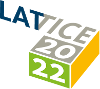#The 39th International Symposium on Lattice Field Theory (Lattice 2022)

Aug 8 – 13, 2022
Hörsaalzentrum Poppelsdorf
Europe/Berlin timezone

## The hadronic running of the electromagnetic coupling and electroweak mixing angle (Part I)

Aug 9, 2022, 5:30 PM
20m
CP1-HSZ/0.001 (CP1-HSZ) - HS3 (CP1-HSZ)

### CP1-HSZ/0.001 (CP1-HSZ) - HS3

#### CP1-HSZ

50
Show room on map
Oral Presentation QCD in searches for physics beyond the Standard Model

### Speaker

Miguel Teseo San José Pérez (IJCLab)

### Description

We employ $N_\mathrm{f}=2+1$ flavours of $O(a)$ improved Wilson fermions to determine the hadronic vacuum polarisation functions $\bar{\Pi}^{\gamma\gamma}$ and $\bar{\Pi}^{\gamma Z}$ for Euclidean squared momenta $Q^2\leq 7$ $\mathrm{GeV}^2$. We extrapolate the results to the physical point using ensembles at four lattice spacings and several pion masses, including its physical value. We observe a tension of up to 3.5 standard deviations between our lattice results for $\Delta\alpha_\mathrm{had}^{(5)}(-Q^2)$ and estimates based on the $R$-ratio for space-like momenta in the range $3-7~\mathrm{GeV}^2$.

### Primary authors

Andreas Risch (DESY, NIC) Miguel Teseo San José Pérez (IJCLab) Antoine Gérardin (Aix-Marseille-Universit ́e, Universit ́e de Toulon, CNRS, CPT, Marseille, France) Georg von Hippel (JGU Mainz) Hartmut Wittig Harvey B. Meyer (Johannes Gutenberg University Mainz) Jonas Wilhelm Kohtaroh Miura (Helmholtz-Institut Mainz, Johannes Gutenberg-Universit ̈at Mainz, Germany) Konstantin Ottnad (University of Mainz) Marco Cè (AEC and ITP, Universität Bern)